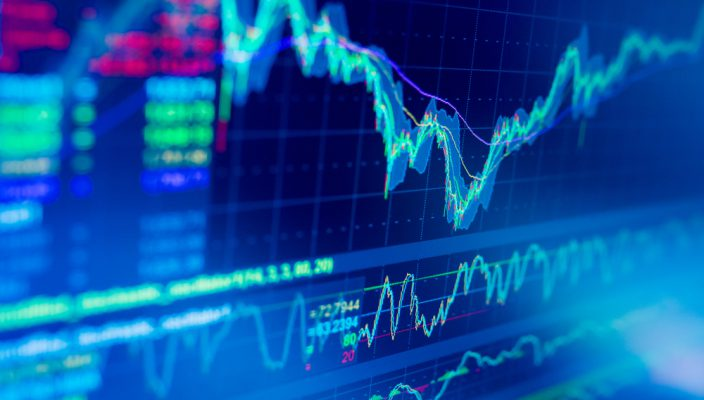## What is Moving Average in Forex?

Apr 21 • Forex Trading Articles • 1291 Views • Comments Off on What is Moving Average in Forex?

Let’s start with the definition. MA is a trend indicator that displays the average price over a specified time interval. The size of this time interval is called a period.

So, a moving average with a period of 200 calculates the average price value based on the last 200 candles, and if the period is 14, then MA will show us the average price value based on the last 14 candles. In other words, period is the number of bars that are taken into account when plotting a line.

## MA types and calculation

You should also understand the method of calculating the moving average. Based on the types, the calculation of moving averages varies slightly.

Simple Moving Average abbreviated SMA – characterized in that the calculation of the same extent takes into account all the candles, starting from the first and ending with the last one.

Exponential Moving Average is abbreviated as EMA. It differs from SMA in that it gives more importance to the last candlestick than the first. So, if we have an exponential moving average with a period of 200 set on the chart, then, candles from 1 to 50 will have the smallest value in the calculation, from 50-100 more important, from 100-150 of medium importance and from 150 to 200 the most important candles that the EMA takes into account. All values ​​are approximate and are taken solely for understanding the general principle.

Next on the list smoothed moving average. In fact, this is a kind of EMA, only the calculation formula is somewhat different. I think it makes no sense to delve so deeply into the technical subtleties, especially since the Smoothed Moving Average is used very rarely in view of the fact that everyone is much more familiar with EMA.

Last in the list is linear weighted moving average. Perhaps it is the most rarely used. It is also a kind of EMA and, in fact, differs only in that it distributes the value of the bars on which it is calculated in a slightly different way.

From this information it is worth considering that the most popular are only 2 moving averages: EMA is the most frequently used, SMA is used less often, but it is also very commonly applied moving average.

It is worth mentioning the “Apply to” option, which is set to Close by default. This parameter is responsible for the data used to construct the MA. Close – at the closing price, Open – at the opening price, High – candle high, Low candle, average price, weighted close. Climbing into these settings without a clear understanding of why is not worth it. Classically, moving averages are built on the closing price, that is, the way it is selected by default and there is nothing to set up here.

## Fast and Slow Moving Averages

The shorter the period, the more sensitively and promptly moving average react to each change in quotes. Therefore, moving averages with small periods are called fast moving average. On the other hand, higher the period of the moving average, the more the MA is sluggish and does not react at all to any small price fluctuations. This is a slow moving average.

There are no clear values ​​at which fast MAs end and slow MAs begin, everything is rather arbitrary. For example, periods up to ~ 25 can be considered fast, from 25 to ~ 50 – the middle, but from 50 and above – slow. Fast MAs just “stick” to the price and follow it on its heels, writing out zigzag forex indicators. Slow ones are rendered much smoother moving average periods.

## Using moving average

There are some trading strategies based on moving averages. For example, if one line crosses another from the bottom up, then this is a buy signal for us, and if on the contrary – from top to bottom, then this is a sell signal. Here the period, which we mentioned earlier, will play a role. Since we have already figured out what fast and slow moving averages are, we can already understand it well.